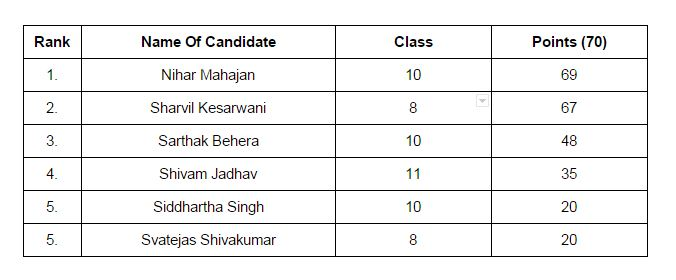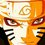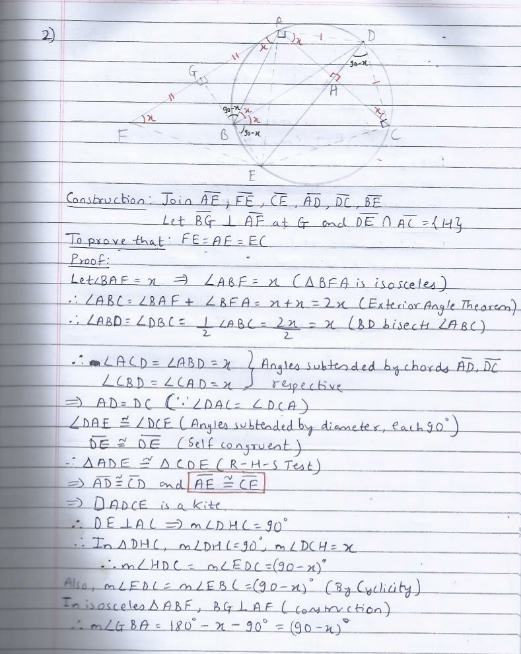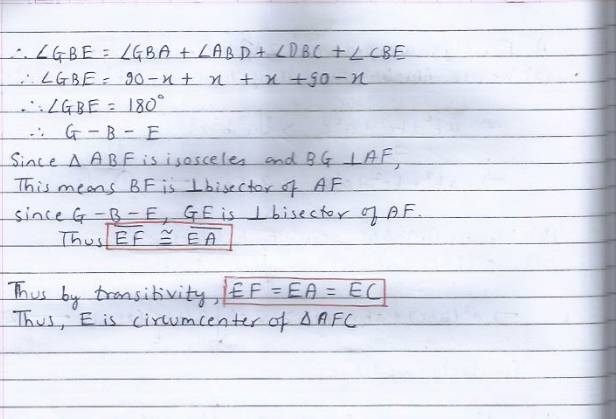# RMO Online Mock Test - Results

So, RMO online mock test is completed.

Only 6 members had sent their solutions. So, here are the results.So, Congratulations Nihar. You stood first.Note by Surya Prakash
5 years, 10 months ago

This discussion board is a place to discuss our Daily Challenges and the math and science related to those challenges. Explanations are more than just a solution — they should explain the steps and thinking strategies that you used to obtain the solution. Comments should further the discussion of math and science.

When posting on Brilliant:

• Use the emojis to react to an explanation, whether you're congratulating a job well done , or just really confused .
• Ask specific questions about the challenge or the steps in somebody's explanation. Well-posed questions can add a lot to the discussion, but posting "I don't understand!" doesn't help anyone.
• Try to contribute something new to the discussion, whether it is an extension, generalization or other idea related to the challenge.

MarkdownAppears as
*italics* or _italics_ italics
**bold** or __bold__ bold
- bulleted- list
• bulleted
• list
1. numbered2. list
1. numbered
2. list
Note: you must add a full line of space before and after lists for them to show up correctly
paragraph 1paragraph 2

paragraph 1

paragraph 2

[example link](https://brilliant.org)example link
> This is a quote
This is a quote
    # I indented these lines
# 4 spaces, and now they show
# up as a code block.

print "hello world"
# I indented these lines
# 4 spaces, and now they show
# up as a code block.

print "hello world"
MathAppears as
Remember to wrap math in $$ ... $$ or $ ... $ to ensure proper formatting.
2 \times 3 $2 \times 3$
2^{34} $2^{34}$
a_{i-1} $a_{i-1}$
\frac{2}{3} $\frac{2}{3}$
\sqrt{2} $\sqrt{2}$
\sum_{i=1}^3 $\sum_{i=1}^3$
\sin \theta $\sin \theta$
\boxed{123} $\boxed{123}$

Sort by:

Names of the ebooks prizes ? ( I guess you won't mind to reveal :) ).

- 5 years, 10 months ago

Hey Guys I will just give you an easy solution to problem 2.

It can be easily observed that $E$ is the midpoint of arc $AC$ containing $B$. Let us drop a perpendicular $N$ from $E$ to on to $BC$. According to Archimedes Broken Chord Theorem, $CN = BN + AB = BN + BF = FN$. So, $N$ is midpoint of $CF$. It implies that $EN$ is perpendicular bisector of $CF$. Now, it is easy to prove that $DE$ is perpendicular bisector of $AC$. As perpendicular bisectors of $CF$ and $AC$ i.e. $EN$ and $ED$ respectively meet at $E$. So, $E$ is the circumcenter of $\Delta AFC$.

Hence Proved.

- 5 years, 10 months ago

It would be better if you even mention proof to Archimedes broken chord.

- 5 years, 10 months ago

All I did was prove congruences and did a bit of angle chasing. My solution is longer but simpler.

- 5 years, 10 months ago

Can you post the solutions to the first and the second questions?@Surya Prakash

- 5 years, 10 months ago

Yes I too want to see the solution for the second one.

- 5 years, 10 months ago

How many of the problems did you get?

- 5 years, 10 months ago

Tried the first 4 got 2.

- 5 years, 10 months ago

I got all 6.

- 5 years, 10 months ago

Thank you everyone :) Also thanks @Surya Prakash for holding this contest. Hope to see more challenging upcoming contests :)

- 5 years, 10 months ago

Congrats! Nihar Mahajan.You did best!

- 5 years, 10 months ago

- 5 years, 10 months ago

Can we get our individual question marks and best solutions for each problem?

- 5 years, 10 months ago

Congratulations! @Nihar Mahajan

- 5 years, 10 months ago

Congratulations Nihar!!

- 5 years, 10 months ago

Congrats nihar but want to ask you did you solve the paper in 3 hrs because I did .

- 5 years, 10 months ago

I did the first 5 questions in 2 hours. The 6th question required time though...

- 5 years, 10 months ago

- 5 years, 10 months ago

Sure.- 5 years, 10 months ago

Can you post solution to 5,6 problem

- 5 years, 10 months ago

Q5)

Extend AI to meet the circumcircle of $\Delta ABC$ at $X$, $BI$ at $Y$ and $CI$ at $Z$. Note that $\angle BAX=\angle BCX$ and $\angle CAX = \angle CBX$ by cyclic bowties (Angles subtended by the same chord). Also, $\angle BAX=\angle CAX$ because $AX$ is the angle bisector of $\angle BAC$. Therefore, $\angle CBX=\angle BCX$, which implies $\Delta BXC$ is an isosceles triangle, which further implies $BX=CX$. Now, we have to prove $BX=IX$.

We have

\begin{aligned} \angle XBI&=\angle XBC+\angle CBI\\ &=\angle XAC+\angle CBI\\ &=\angle IAC+\angle CBI\\ &=\dfrac {1}{2} \angle BAC + \dfrac {1}{2} \angle ABC \end{aligned}

Also,

\begin{aligned} \angle XIB&=\angle IAB+ \angle IBA \text{(}\angle XIB \text{ is the external angle of } \Delta AIB\text{)}\\ &=\dfrac{1}{2} \angle BAC+\dfrac{1}{2} \angle ABC \end{aligned}

Thus, $\angle XBI=\angle XIB$, which implies $\Delta XIB$ is isosceles, which implies $XI=XB$. We already know that $XB=XC$, so $X$ is the circumcentre of $\Delta IBC$. By definition, point $X$ is on the circumcircle of $\Delta ABC$. Therefore, the circumcentre of $\Delta IBC$ lies on the circumcircle of $\Delta ABC$. Similarly, it can be proven that the circumcentre ($Y$) of $\Delta ICA$ lies on the circumcircle of $\Delta ABC$ and the circumcentre ($Z$) of $\Delta IAB$ lies on the circumcircle of $\Delta ABC$.

- 5 years, 10 months ago

I would not recommend my solution for Q6 since it is (a) 4 pages long and (b) Proves a massive generalisation.

- 5 years, 10 months ago

What!Really?!My solution is a page long!

- 5 years, 10 months agoQ6,Q3

- 5 years, 10 months ago

@Shivam Jadhavcould you post the solution to the first one?

- 5 years, 10 months ago

$ab(a+b)+ac(a+c)+bc(b+c)= \dfrac{ab(a+b)+ac(a+c)+bc(b+c)+ab(a+b)+ac(a+c)+bc(b+c)}{2}=\dfrac{a^2(b+c)+b^2(a+c)+c^2(a+b)+(a^2b+b^2c+a^2c)+(ab^2+bc^2+ac^2)}{2}$

By AM-GM inequality, $ab^2+bc^2+ac^2 \ge 3abc$

Therefore,

$\dfrac{a^2(b+c)+b^2(a+c)+c^2(a+b)+(a^2b+b^2c+a^2c)+(ab^2+bc^2+ac^2)}{2} \ge \dfrac{a^2(b+c)+b^2(a+c)+c^2(a+b)+(a^2b+b^2c+a^2c)+3abc}{2}=\dfrac{a^2(b+c)+b^2(a+c)+c^2(a+b)+ab(a+c)+bc(a+b)+ac(b+c)}{2}$

Grouping and again applying AM-GM inequality, (Applying AM-GM inequality on each term and adding all the terms)

$\dfrac{(a^2(b+c)+ab(a+c))}{2}+\dfrac{(b^2(a+c)+bc(a+b))+}{2}+\dfrac{(c^2(a+b)+ac(b+c))}{2} \ge \sqrt{a^3b(b+c)(a+c)}+\sqrt{b^3c(a+c)(a+b)}+\sqrt{c^3a(a+b)(a+c)}=\displaystyle\sum_{cyc}ab\sqrt{\dfrac{a}{b}(b+c)(c+a)}.$ Equality occurs when $a=b=c$

Hence proved.

- 5 years, 10 months ago

Woah!That is a big solution!Could you write the motivation please?

- 5 years, 10 months ago

Congratulations @Nihar Mahajan !

- 5 years, 10 months ago

@Surya Prakash Thank you very much for holding the content. When will the second one start?

- 5 years, 10 months ago

I can't say when because I am somewhat busy with preparation for NSEP.

- 5 years, 10 months ago

I also want to participate insuch contests . Can any one suggest me the pathway and dates for future such contests

- 5 years, 10 months ago

Thanks! to Surya Prakash for this opportunity.

- 5 years, 10 months ago

Hey guys. The ebooks i.e. prizes are sent to the participants. Please check your mails.

- 5 years, 10 months ago

When was it held?

- 5 years, 9 months ago

@Surya Prakash Could u please conduct an INMO mock test similar to this ?

- 5 years, 7 months ago